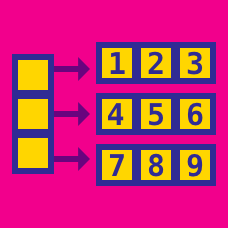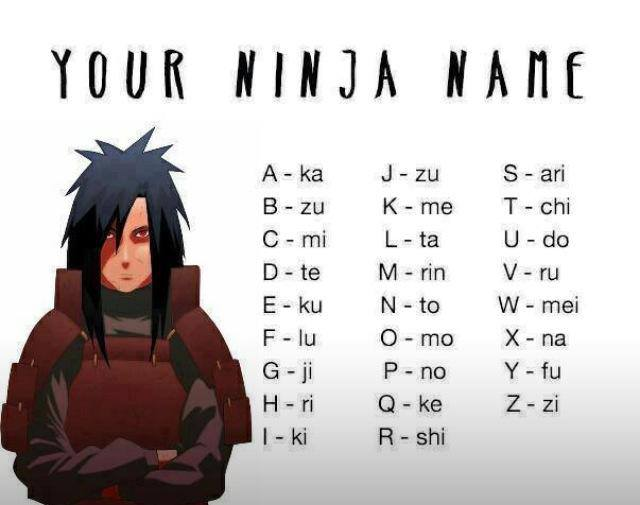Computer Science

# Linear Data Structures: Level 3 ChallengesUsing the above image, one would can Ninja-encode a phrase by replacing each letter by the corresponding phrase.

For example, my name (Lokesh) came out to be 'ta~mo~me~ku~ari~ri'. Notice, I have placed '~' among the sub-words to make the reverse translation easier. I will follow the same notation while converting any other word to Ninja name.

Now, write a program that converts any name in English to Ninja name. Solve this puzzle and enter the answer below

  1 2 3 4 5 6 7 8 9 10 chi~ri~ku ka~to~ari~mei~ku~shi chi~mo chi~ri~ki~ari no~shi~mo~zu~ta~ku~rin ki~ari ari~ki~na te~ki~ru~ki~te~ku~te zu~fu ri~ka~ta~lu 

Given an array and an element $x$, the floor of an element $x$ is defined as the greatest element present in the array which is less than or equal to $x$.

What is the worst case complexity of the most efficient algorithm for finding a floor of an element $x$ in a sorted array?

Details and Assumptions:

• If the array is $[3, 8, 15, 19, 23]$ and $x=20$, then the output will be $19$.

• $x$ can't be less than the minimum element in the list.Given a singly linked list of size $n>10$ what is the time complexity of finding the 10th element form the front and the 10th element from the end respectively?

##### Image Credit: Wikipedia.
×

Problem Loading...

Note Loading...

Set Loading...